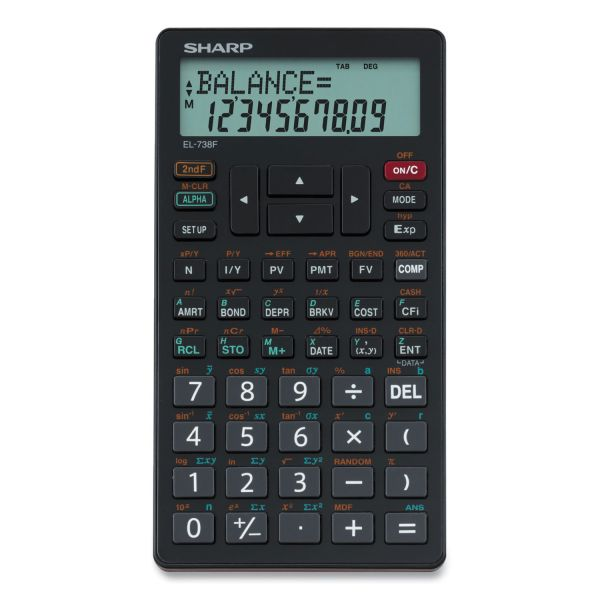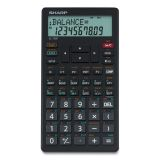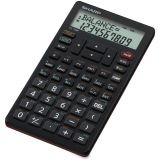# Sharp EL-738C Financial Calculator, 10-Digit LCD

Product Number: SHREL738FB
• Large 10 Digit, 2 Line LCD Display
• Time Value Money Solver
• Amortization Calculation
• Cash Flow Analysis, NPR, IRR
More details
Sold Out

This product is sold out and we do not know when we will be getting another shipment. We apologize for any inconvenience this may cause.

Check similar items above to see if a replacement product is available.

## Product Description

Sharp EL-738C Financial Calculator, 10-Digit LCD - This 10-digit financial calculator computes all time-value-of-money calculations. Large, 10-digit, liquid crystal display (LCD) features two lines and mode indicators. Functions include amortization calculations and interest rate conversions, nonuniform cash flow calculations, two-variable statistics with linear regression, cost/sell/margin and markup calculations, sign change, percent and square root. Lightweight calculator weighs 3.5 oz. and includes batteries and a wallet cover with pocket.

• Large 10 Digit, 2 Line LCD Display
• Time Value Money Solver
• Amortization Calculation
• Cash Flow Analysis, NPR, IRR
• Depreciation with Three Different Methodologies
• Breakeven, Profit and Percent Difference Calculations
• 2nd Key Feature to Calculate Terms Fast
Guides & Information
Related Articles

### Product Specifications

• +/- Switch Key
Yes
• Amortization
Yes
• Assembly Required
No
• Backspace Key
Yes
• Base Number Calculations
No
• Bond Calculations
No
• Brand Name
Sharp Calculators
• Calculator Features
2-line Display Independent Memory Large LCD
• Case
Wallet
• Complex Number Calculations
No
• Confidence Interval Calculating
No
• Cost/Sell/Margin
Yes
• Country of Origin
China
• Currency Exchange Function
No
• Date Calculations
No
• Decimal Function
No
• Depreciation Calculations
No
• Depth
5.9"
• Display Angle
Fixed
• Display Characters Height
12 mm
• Display Notation
Numeric
• Display Screen Type
Dot Matrix
• Display Type(s)
LCD
• Double Zero Key
No
• Environmentally Friendly
Yes
• Equation Editor
No
• Fraction/Decimal Conversions
No
• Fraction Calculations
No
• Global Product Type
Calculators-Financial
• Grand Total Key
No
• Height
0.4"
• Hyperbolic Functions
No
• Hypothesis Testing
No
• Interest Rate Conversion
Yes
• Item Count Function
No
• Linear Regression
No
• Loan Calculation
Yes
• Logical (Boolean) Operations
No
• Manufacturer Part Number
EL-738FB
• Markup/Down Key
No
• Matrices
No
• Metric Conversion
No
• Number of Display Digits
10
• Number of Display Lines
2
• Package Contents
Advanced Financial Calculator with Scientific Functions Protective Slide Cover
No
• Percent Key(s)
No
• Polar-Rectangular Conversion
No
• Post-Consumer Recycled Content Percent
0%
• Post-consumer-waste%
0%
• Power Source
Battery
• Power Source(s)
Battery
• Pre-Consumer Recycled Content Percent
0%
• Probability (Random Number)
No
• Product Model
EL738FB
• Product Name
Advanced Financial Calculator with Scientific Functions
• Product Type
• Recycled
No
• Replacement Batteries
CR2032
• Simultaneous Equations
No
• Size
3 1/5 x 5 9/10
• Square Root Key
No
• Tax Calculation
No
• Time-Value-of-Money
Yes
• Time/Date
No
• Total Recycled Content Percent
0%
• Trig/Log Functions
No
• Variable Regression
No
• Width
3.3"